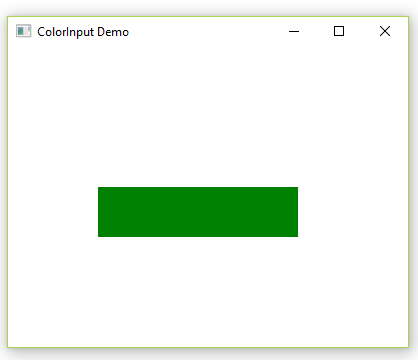# JavaFX | ColorInput Class

ColorInput class is a part of JavaFX. It is used to create an effect which renders a rectangular region, filled with the given Color. It is equivalent to rendering a filled rectangle into an image and using an ImageInput effect, except that it is more convenient and potentially much more efficient. It is mainly passed into the other effects as an input.

Constructors of the class:

1. ColorInput(): Creates a new instance of ColorInput with default parameters.
2. ColorInput(double x, double y, double width, double height, Paint paint): Creates a new instance of ColorInput with the specified x, y, width, height, and paint.

Commonly Used Methods:

Method Description
getX() Gets the value of the property x.
getY() Gets the value of the property y.
setHeight(double value) Sets the value of the property height
setPaint(Paint value) Sets the value of the property paint.
setWidth(double value) Sets the value of the property width.
setX(double value) Sets the value of the property x.
setY(double value) Sets the value of the property y.
getHeight() Gets the value of the property height.
getPaint() Gets the value of the property paint.
getWidth() Gets the value of the property width
1. Java program to Demonstrate ColorInput class: In this program, ColorInput Effect is created and then we set the color, height, width, and coordinates of the region of ColorInput. A group object and scene object is created. A scene is added to the stage and then we set the title of the stage.

 `// Java program to Demonstrate ColorInput class ` `import` `javafx.application.Application; ` `import` `javafx.scene.Group; ` `import` `javafx.scene.Scene; ` `import` `javafx.scene.effect.ColorInput; ` `import` `javafx.scene.paint.Color; ` `import` `javafx.scene.shape.Rectangle; ` `import` `javafx.stage.Stage; ` ` `  `public` `class` `ColorInputDemo ``extends` `Application { ` ` `  `    ``// Main Method  ` `    ``public` `static` `void` `main(String[] args) ` `    ``{ ` ` `  `        ``// launch the application ` `        ``launch(args); ` `    ``} ` ` `  `    ``// launch the application ` `    ``public` `void` `start(Stage primaryStage) ``throws` `Exception ` `    ``{ ` ` `  `        ``// Instantiating the ColorInput class ` `        ``ColorInput color = ``new` `ColorInput(); ` ` `  `        ``// set the color ` `        ``color.setPaint(Color.GREEN); ` ` `  `        ``// sets the height of the region of color input ` `        ``color.setHeight(``50``); ` ` `  `        ``// sets the width of the region of color input ` `        ``color.setWidth(``200``); ` ` `  `        ``// set the coordinates of the Colorinput ` `        ``color.setX(``90``); ` `        ``color.setY(``140``); ` ` `  `        ``// create a rectangle ` `        ``Rectangle rect = ``new` `Rectangle(); ` ` `  `        ``// applying coloradjust effect ` `        ``rect.setEffect(color); ` ` `  `        ``// create a group object ` `        ``Group root = ``new` `Group(); ` ` `  `        ``// create a scene object ` `        ``Scene scene = ``new` `Scene(root, ``400``, ``300``); ` ` `  `        ``root.getChildren().add(rect); ` ` `  `        ``// adding scene to the stage ` `        ``primaryStage.setScene(scene); ` ` `  `        ``// set title of the stage ` `        ``primaryStage.setTitle(``"ColorInput Demo"``); ` ` `  `        ``primaryStage.show(); ` `    ``} ` `} `

Output:2. Java program to apply ColorInput class to the created rectangle by clicking on the button using EventHandler: In this program, we first set the Height, Width, and coordinates of a rectangle and then create a rectangle of the same dimension. Now, create a Button and set the Layouts of the Button. Now, using EventHandler, first, instantiate a ColorInput class using proper dimension and then set ColorInput Effect to the Button. Create a group object and add Button and rectangle to it. Then Create a Scene and add it to the stage.

 `// Java program to apply ColorInput class to  ` `// the created rectangle by clicking on the  ` `// button using EventHandler ` `import` `javafx.application.Application; ` `import` `javafx.event.ActionEvent; ` `import` `javafx.event.EventHandler; ` `import` `javafx.scene.Group; ` `import` `javafx.scene.Scene; ` `import` `javafx.scene.control.Button; ` `import` `javafx.scene.effect.ColorInput; ` `import` `javafx.scene.paint.Color; ` `import` `javafx.scene.shape.Rectangle; ` `import` `javafx.stage.Stage; ` ` `  `public` `class` `ColorInputExample ``extends` `Application { ` ` `  `    ``public` `void` `start(Stage stage) ` `    ``{ ` ` `  `        ``double` `x = ``10``; ` `        ``double` `y = ``10``; ` `        ``double` `w = ``40``; ` `        ``double` `h = ``180``; ` ` `  `        ``// Rectangle ` `        ``Rectangle rect = ``new` `Rectangle(x, y, w, h); ` `        ``rect.setFill(Color.WHITE); ` `        ``rect.setStrokeWidth(``1``); ` `        ``rect.setStroke(Color.BLACK); ` ` `  `        ``// Button ` `        ``Button button = ``new` `Button(``"Click To See the Effects!"``); ` ` `  `        ``// set button layout coordinates ` `        ``button.setLayoutX(``100``); ` `        ``button.setLayoutY(``30``); ` ` `  `        ``button.setPrefSize(``250``, ``150``); ` ` `  `        ``button.setOnAction(``new` `EventHandler() { ` ` `  `            ``public` `void` `handle(ActionEvent event) ` `            ``{ ` `                ``// instantiating the colorinput class ` `                ``ColorInput colorInput = ``new` `ColorInput(x, y,  ` `                                     ``w, h, Color.STEELBLUE); ` ` `  `                ``// Setting ColorInput effect ` `                ``button.setEffect(colorInput); ` `            ``} ` `        ``}); ` ` `  `        ``// create the Group object ` `        ``Group root = ``new` `Group(); ` ` `  `        ``root.getChildren().addAll(button, rect); ` ` `  `        ``Scene scene = ``new` `Scene(root, ``450``, ``300``); ` `        ``stage.setTitle(``"JavaFX ColorInput Effect"``); ` `        ``stage.setScene(scene); ` ` `  `        ``stage.show(); ` `    ``} ` ` `  `    ``// Main Method ` `    ``public` `static` `void` `main(String args[]) ` `    ``{ ` ` `  `        ``// Launch the application ` `        ``launch(args); ` `    ``} ` `} `

Output:

Note: The above programs might not run in an online IDE. Please use an offline compiler.My Personal Notes arrow_drop_upCheck out this Author's contributed articles.

If you like GeeksforGeeks and would like to contribute, you can also write an article using contribute.geeksforgeeks.org or mail your article to contribute@geeksforgeeks.org. See your article appearing on the GeeksforGeeks main page and help other Geeks.

Please Improve this article if you find anything incorrect by clicking on the "Improve Article" button below.

Article Tags :
Practice Tags :

1

Please write to us at contribute@geeksforgeeks.org to report any issue with the above content.